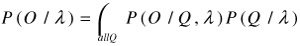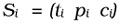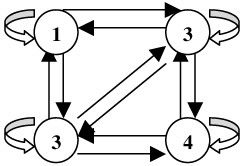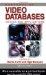# 5. SceneStory Boundary Detection

## 5. Scene/Story Boundary Detection

After the shots have been classified into one of the pre-defined categories, we employ HMMs to detect scene/story boundaries. We use the shot sequencing information, and examine both the tagged categories and appropriate features of the shots to perform the analysis. This is similar to the idea of part-of-speech (POS) tagging problem in NLP that uses a combination of POS tags and lexical information to perform the analysis.

HMM is a powerful statistical tool first successfully utilized in speech recognition research. HMM contains a finite set of states, each of which is associated with a probability distribution. Transitions among the states are governed by a set of probabilities called transition probabilities. In a particular state, an outcome or observation can be generated according to the associated probability distribution. We can express the HMM parameters as the following. Each HMM is modelled with a set λ = (π, A, B). Here, π = (π1,, πN) is the initial state probability; and N is the number of states represented by Q = {1, 2, ...,N}. A = {aij} is the state transition probability matrix where 1 i N and 1 j N; and aij is the probability of moving from state i to state j. B = {bjk} is the observation probability distribution matrix with 1 j N and 1 k M; bjk is the emission of symbol k at state j; and M is the number of symbols. Finally, V is the set of symbols (feature vectors), with V = {v1, v2 , v3vM}. Given a HMM λ, and the observation sequence O = (O1, O2, OT), P(O|λ) is the probability that HMM λ produces the observation sequence O. P(O|λ) can be computed by:

 (44.2)Further details on HMM can be found in .

In our approach, we represent each shot by: (a) its tagged category; (b) scene/location change (c= change, u = unchanged); and, (c) speaker change (c = change, u = unchanged). We use the tag id as defined in Figure 44.3 to denote the category of each shot. The commercial category is not used here, so there are 12 categories. Each shot i is thus represented by a feature vector given by:

 (44.3)where ti is the tag id of shot i; pi is the speaker change indicator (c or u); and ci is the scene change indicator (c or u). Thus, each output symbol is represented by 1 of the 12 possible categories of shots, 1 out of 2 possible scene change feature, and 1 out of 2 possible speaker change feature. This gives a total of 12x2x2 = 48 distinct vectors for modeling using the HMM framework.

In our priliminary experiments, we employ the ergodic HMM framework. We perform the experiments by varying the number of states from 4 to 9 to evaluate the results. As we have a small training data set, our initial test indicates that the number of state equals to 4 gives the best result. Figure 44.6 illustrates an ergodic HMM with 4 states in our approach. When 4 states are used, we need to estimate bjk for 4x48 = 192 probabilities.Figure 44.6: The ergodic HMM with 4 hidden statesHandbook of Video Databases: Design and Applications (Internet and Communications)
ISBN: 084937006X
EAN: 2147483647
Year: 2003
Pages: 393